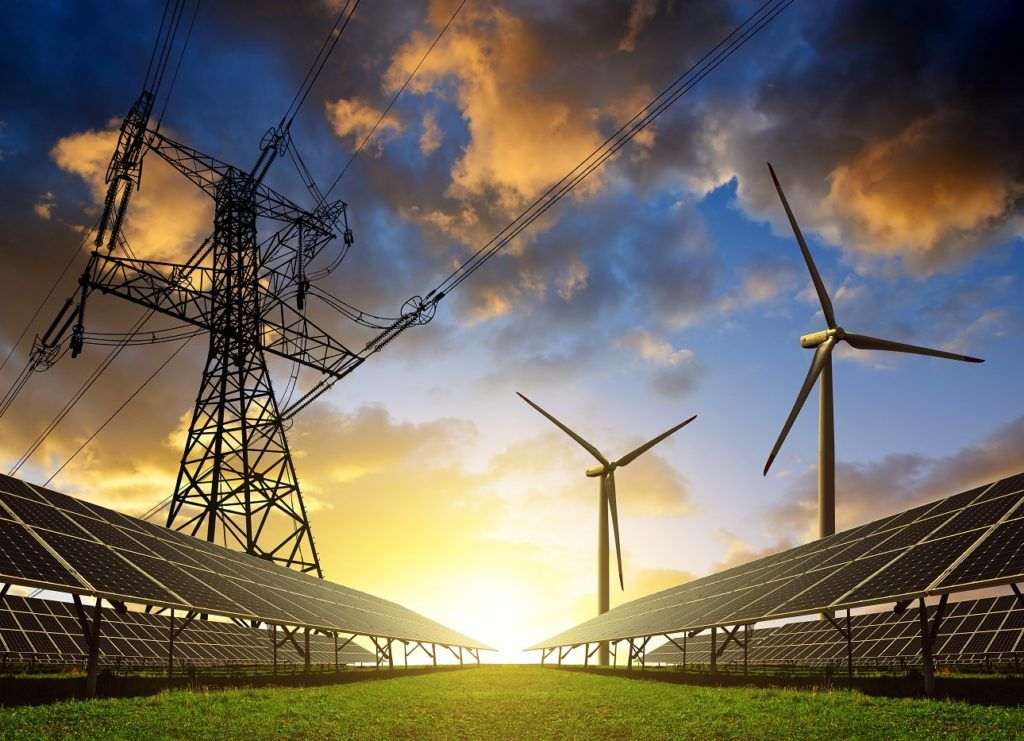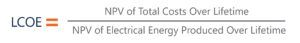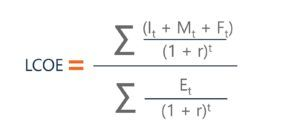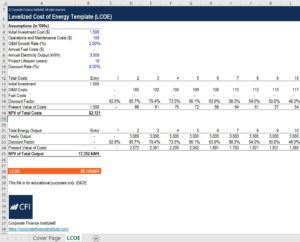# Levelized Cost of Energy (LCOE)

The Average Total Cost of a Project Per Unit of Total Electricity Generated

## What is the Levelized Cost of Energy (LCOE)?

The levelized cost of energy (LCOE), also referred to as the levelized cost of electricity or the levelized energy cost (LEC), is a measurement used to assess and compare alternative methods of energy production. The LCOE of an energy-generating asset can be thought of as the average total cost of building and operating the asset, per unit of total electricity generated over an assumed lifetime.Alternatively, the LCOE can be thought of as the average minimum price in which the electricity generated by the asset is required to be sold at, in order to offset the total costs of production over its lifetime. Calculating the LCOE is related to the concept of assessing a project’s net present value. Similarly to using NPV, the LCOE can be used to determine whether a project will be a worthwhile venture.

### Quick Summary of Points

• The LCOE is a fundamental calculation used in the preliminary assessment of an energy producing project
• The LCOE can be used to both determine whether to move forward with a project or to compare different energy producing projects
• The formula to calculate the LCOE is: (Present Value of Total Cost Over the Lifetime)/(Present Value of All Electricity Generated Over the Lifetime)

### Why is the Levelized Cost of Energy (LCOE) Important?

The LCOE is a very important metric in determining whether or not to move forward with a project. The LCOE will determine if a project will break even, and if it does not, then the firm will not go ahead with building the power-generating asset and will look for an alternative. Using the LCOE to assess a project is one of the first, fundamental steps taken in analyzing projects of this nature.

The LCOE is also an important calculation to allow financial analysts to compare different energy producing technologies such as wind, solar, and nuclear power sources. It allows for these comparisons regardless of unequal life spans, differing capital costs, size of the projects, as well as the differing risk associated with each of the projects. This is because the LCOE is taken as a per unit cost of electricity generated and the risk of each project is an implication of the specific discount rate used for each power-generating asset.

### How to Calculate the Levelized Cost of Energy?

The LCOE can be calculated by first taking the net present value of the total cost of building and operating the power generating asset. This number is then divided by the total electricity generation over its lifetime. The total costs associated with the project generally will include:

• The initial cost of investment expenditures (I)
• Maintenance and operations expenditures (M)
• Fuel expenditures (if applicable) (F)

The total output of the power-generating asset will include:

• The sum of all electricity generated (E)

The last two important factors to be considered in the equation are:

• The discount rate of the project (r)
• The life of the system (n)LCOE = Σ[(It + Mt + Ft) / (1 + r)t] / Σ[(Et/(1 + r)t]

### Levelized Cost of Energy – Worked Example

Let us take a look at a simple levelized cost of energy example question. A hypothetical wind turbine takes 1 year to be built and costs \$1.5 million. The operating and maintenance costs are \$300,000 per year with an associated growth rate of 2% annually. There are no associated fuel costs. The wind turbine’s lifespan is 10 years, and it is estimated to produce 3 million kWh each year. Finally, the associated discount rate for the project is 8%.

The following image shows the calculation of the LCOE of the project. In a comparative analysis, the figure would be calculated for numerous energy sources and compared against one another.To download this free Excel calculator, check out the CFI Marketplace: Levelized Cost of Energy (LCOE) Calculator

### Lazard’s Levelized Cost of Energy Analysis

Lazard Ltd is a financial advisory and asset management firm. Near the end of each year, Lazard releases a levelized cost of energy analysis. At the point in time of this article, they are currently on their twelfth edition. This comparative analysis assesses several forms of energy generation, including:

• Various Solar Technologies
• Fuel Cells
• Geothermal
• Wind
• Gas
• Nuclear
• Coal

If you would like to read Lazard’s analysis, check out Lazard’s Levelized Cost of Energy Analysis – Version 12.0

Thank you for reading CFI’s article on the levelized cost of energy. If you would like to learn more about related concepts, check out CFI’s other resources:

• Net Present Value
• Discount Rate
• Discounted Payback Period
• Financial Modeling of a Wind Project

### Financial Analyst Training

Get world-class financial training with CFI’s online certified financial analyst training program!

Gain the confidence you need to move up the ladder in a high powered corporate finance career path.

Learn financial modeling and valuation in Excel the easy way, with step-by-step training.